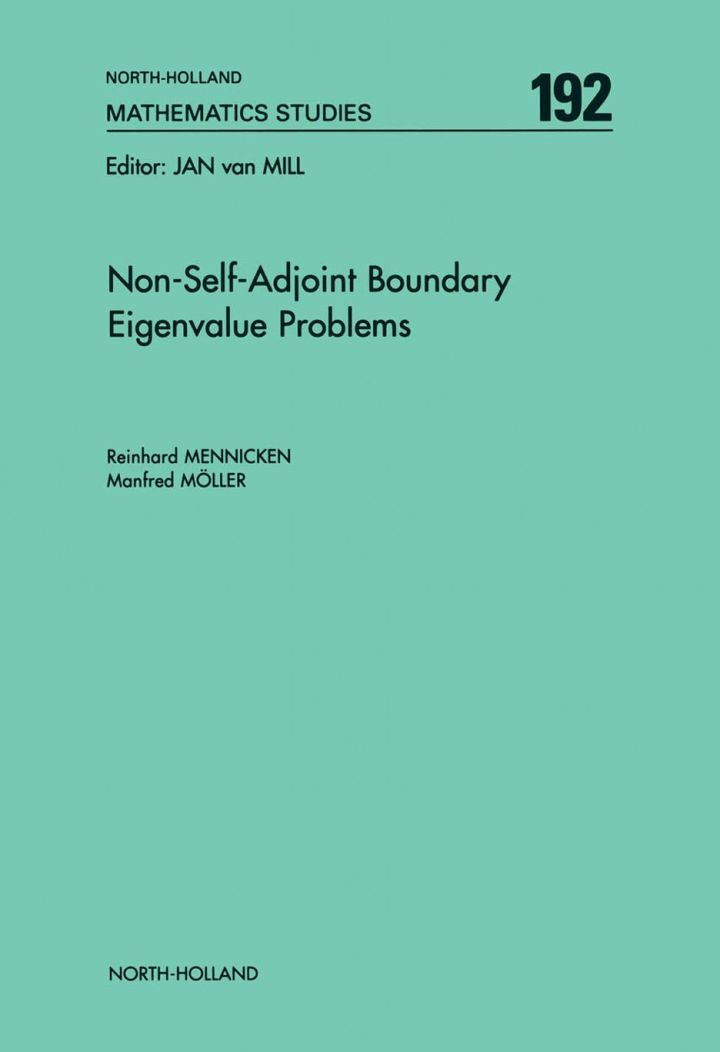# Non-Self-Adjoint Boundary Eigenvalue Problems

## Non-Self-Adjoint Boundary Eigenvalue Problems

By Mennicken, R.; Möller, M.

### Rent or Buy eTextbook

Expires on Apr 14th, 2022
\$225

Publisher List Price: \$229.99
Savings: \$4.99

This monograph provides a comprehensive treatment of expansion theorems for regular systems of first order differential equations and n-th order ordinary differential equations.
In 10 chapters and one appendix, it provides a comprehensive treatment from abstract foundations to applications in physics and engineering. The focus is on non-self-adjoint problems. Bounded operators are associated to these problems, and Chapter 1 provides an in depth investigation of eigenfunctions and associated functions for bounded Fredholm valued operators in Banach spaces. Since every n-th order differential equation is equivalent
to a first order system, the main techniques are developed for systems. Asymptotic fundamental
systems are derived for a large class of systems of differential equations. Together with boundary
conditions, which may depend polynomially on the eigenvalue parameter, this leads to the definition of Birkhoff and Stone regular eigenvalue problems. An effort is made to make the conditions relatively easy verifiable; this is illustrated with several applications in chapter 10.
The contour integral method and estimates of the resolvent are used to prove expansion theorems.
For Stone regular problems, not all functions are expandable, and again relatively easy verifiable
conditions are given, in terms of auxiliary boundary conditions, for functions to be expandable.
Chapter 10 deals exclusively with applications; in nine sections, various concrete problems such as
the Orr-Sommerfeld equation, control of multiple beams, and an example from meteorology are investigated.

Key features:

• Expansion Theorems for Ordinary Differential Equations
• Discusses Applications to Problems from Physics and Engineering
• Thorough Investigation of Asymptotic Fundamental Matrices and Systems
• Provides a Comprehensive Treatment
• Uses the Contour Integral Method
• Represents the Problems as Bounded Operators
• Investigates Canonical Systems of Eigen- and Associated Vectors for Operator Functions

Subject: Mathematics & Statistics -> Mathematics -> Mathematics GeneralPublisher: Elsevier S & T 06/2003
Imprint: North Holland
Language: English
Length: 518 pages

ISBN 10: 0444514473
ISBN 13: 9780444514479
Print ISBN: 9780444514479

Live Chats